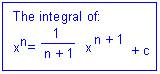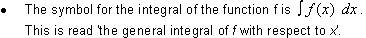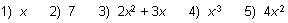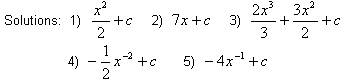# Introduction to Integration - Calculus math review

math tutorials > introduction to integration

## What is integration? A definition of integration.

Integration is the process of finding the definite or indefinite integral of a function.  Integration is the inverse of differentiation and is often called antidifferentiation.c is any fixed number and is called the constant of integration.## Note:

• The result of integrating may be checked by differentiating your answer.
• The integral of 4 = 4x + c## Integration problems for you to try.

Integrate each of the following: